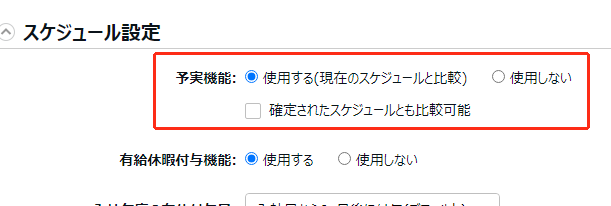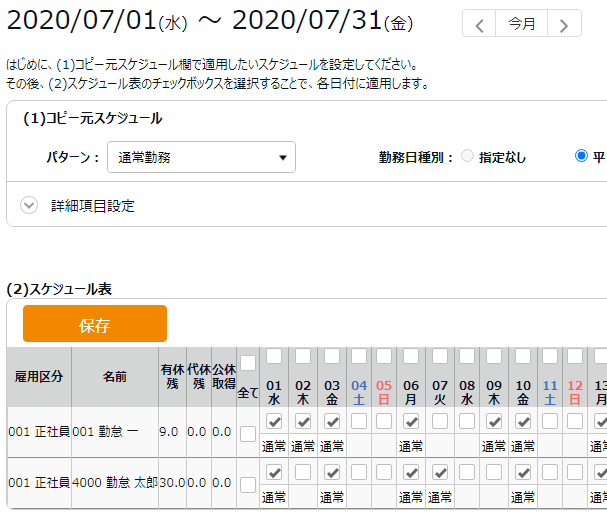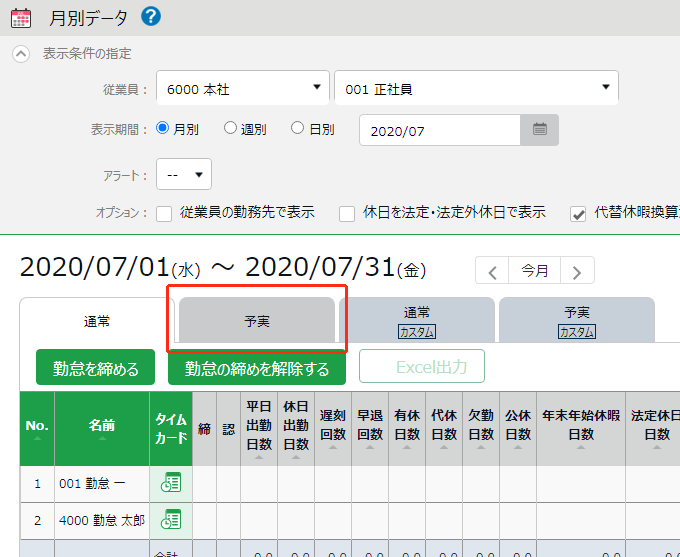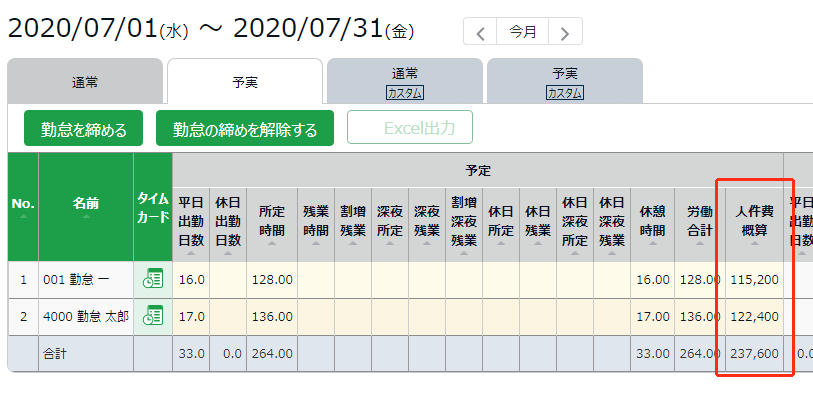# What is the "Labor cost estimate output function"?

◆ Settings "Others" ＞ Options ＞ " Attendance management settings" ＞ "Labor cost estimate output function"

In this field, select whether to calculate personnel cost for time results. You can select either "JPY display (integral numbers)" or "DOL display (up to 2nd decimal)" as the display format.

When calculating personnel costs, you can set unit costs and transportation costs for each employee type and employee. Based on that, you will be able to check the approximate personnel expenses on the daily data and monthly data screens.

• A [Wage] setting button is displayed in the employee type settings and employee settings.
• On this screen screen, the approximate labor cost will be displayed (daily data, monthly data, yearly data).
• It is possible to export approximate personnel costs (monthly data [CSV], daily data [CSV]).

### "Labor cost estimate" calculation method for each day

First, work hours are in minutes.

Example: 1 hour 25 minutes → 85 minutes

The formula is " hourly wage x working hours (minutes) ÷ 60 ". When a decimal point occurs, the first decimal place is rounded off.

1000 yen (hourly wage) × 85 minutes (working time) ÷ 60 = 1,416.66666……

Round off the first decimal place → 1,417 yen

### Calculation method of "Labor cost estimate" for 1 month

Use the above method to calculate the "Labor cost estimate" for each day. However, it does not round to the nearest whole number. If the decimal numbers are infinite, look up to 15 digits after the decimal point.

Example: 1,416.6666666666666666666666……

→ 1,416.666666666666666666 (up to 15 decimal places)

We will continue to add the "Estimated Personnel Expenses" for each day while leaving the decimals.

02/01 1,416.666666666666666

02/02 1,624.5

02/03 1,245.32

→ Total 4,286.486666666666666666

Finally, the first decimal place is rounded off.

4,286.486666666666666 Yen

→ 4,286 yen

`Precautions when selecting the dollar display-Up to 2 digits after the decimal point can be entered when setting the unit price.・If there is a fraction in the labor cost estimate, the figures will be rounded to the second decimal place.`

## Scheduled personnel expenses

When making a shift, I think that there are times when personnel expenses are taken into consideration. You can check the planned personnel cost based on the registered schedule by using the "prediction function".

1. Turn on the prediction function. Settings ＞ Other ＞ Options ＞ "Schedule Settings" ＞ "Plan/actual functions: Apply" ＞ Save2. Assign the schedule.3. Click Home screen> Frequently used menu> “Monthly data”> [Plan/Actual results] tab.4. A prediction field with a yellow background is displayed. "Labor cost estimate" displayed here is the planned personnel cost calculated based on the schedule.In the [Daily Data] screen, you can also check the labor cost for each day on the [Plan/Actual results] tab.